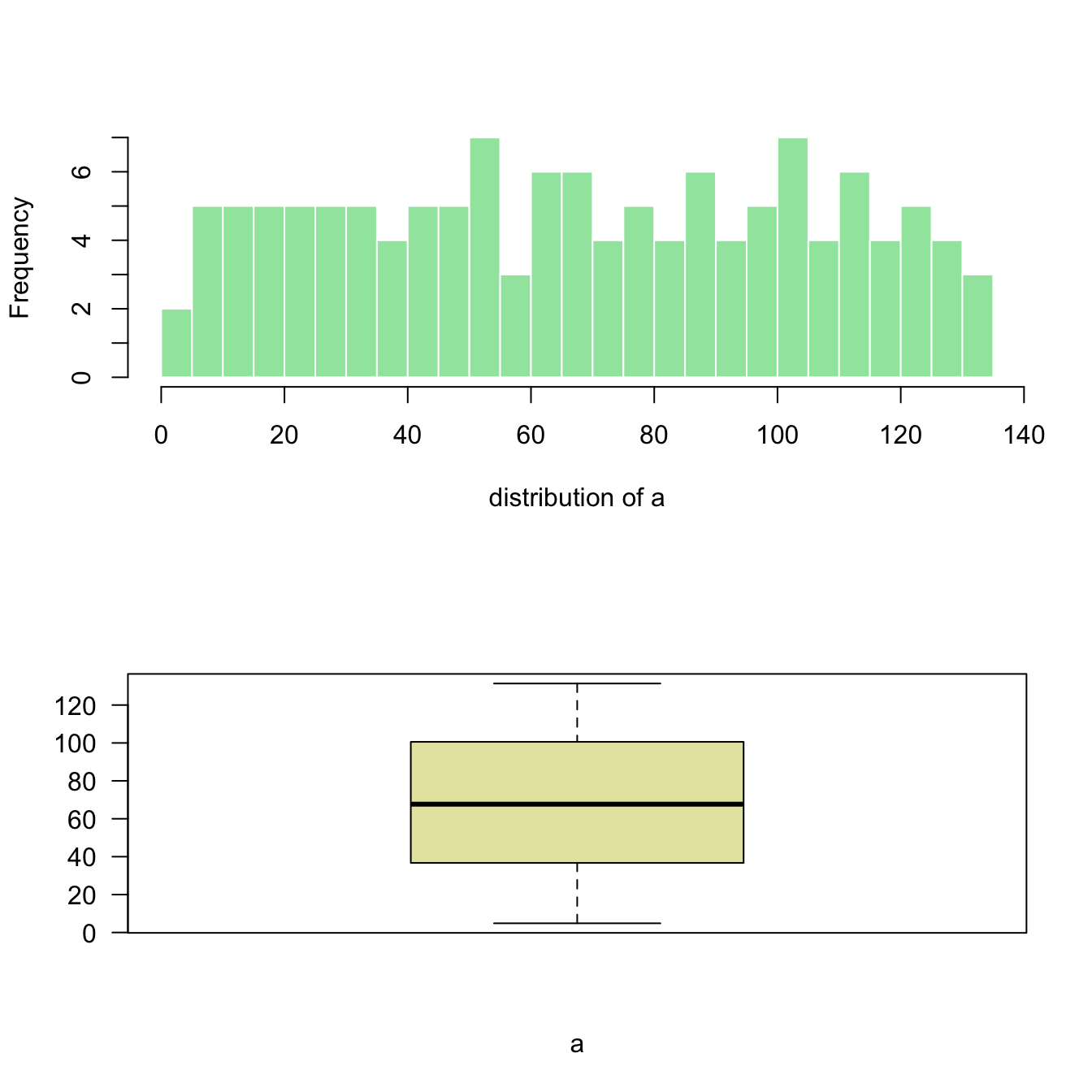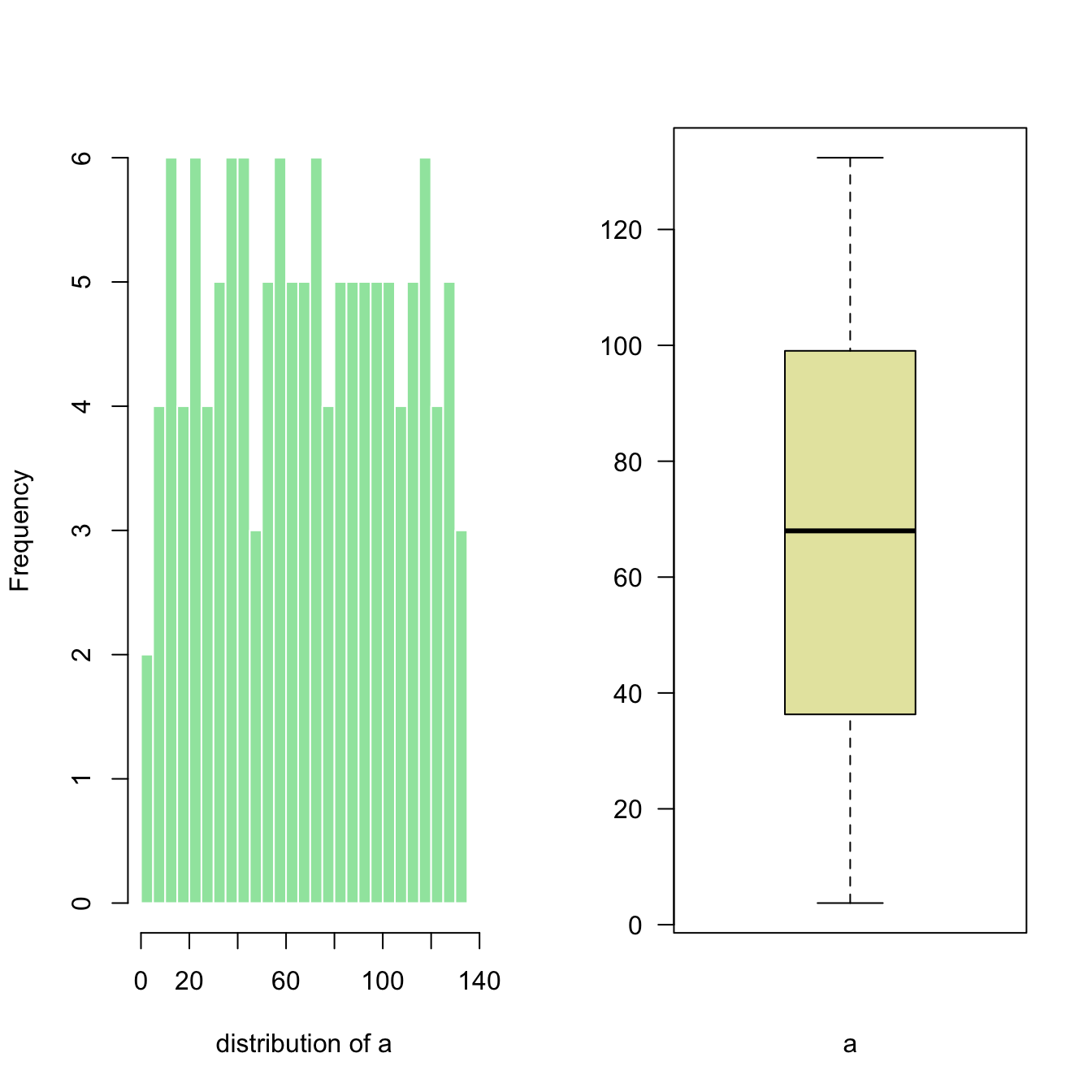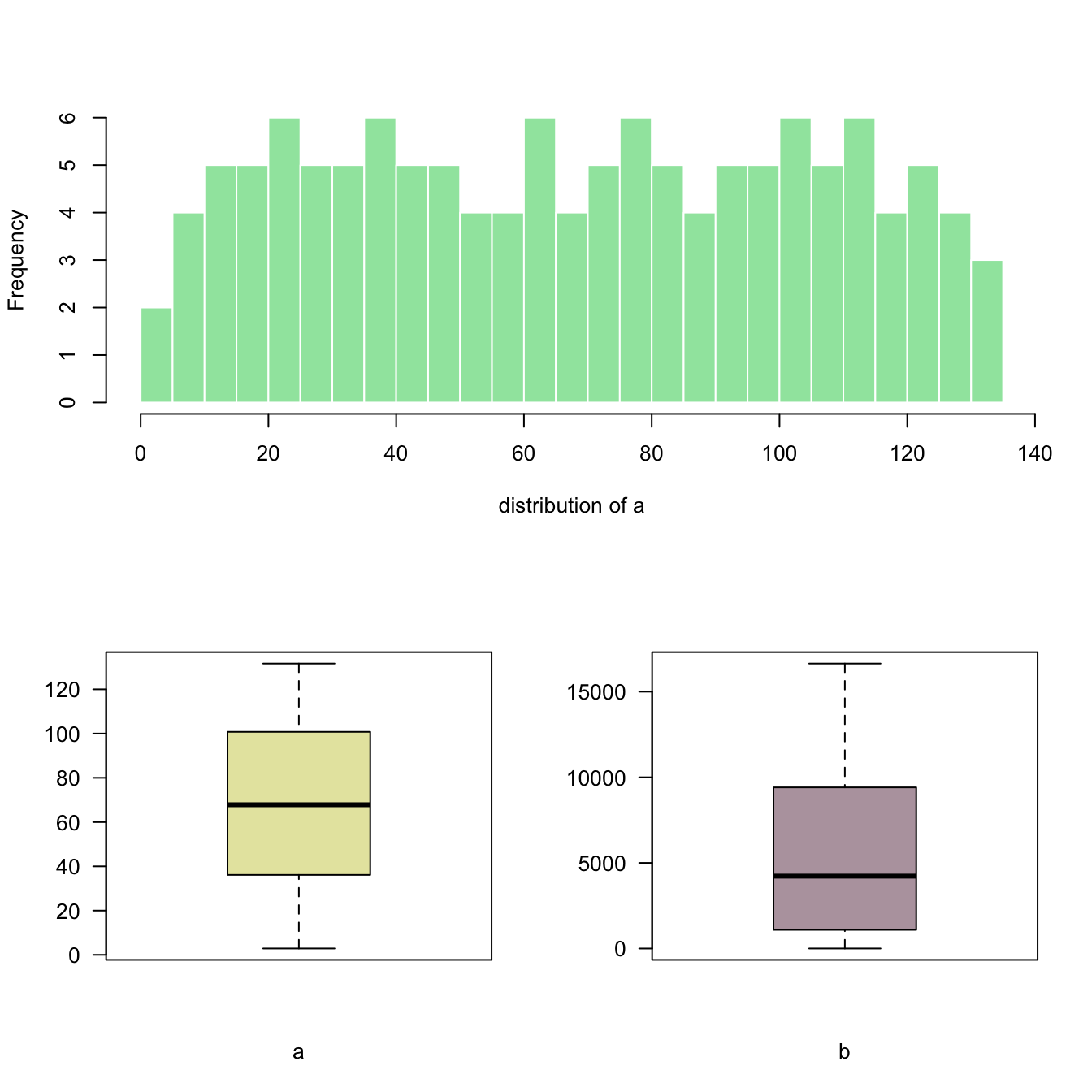# Split base R plot window with layout()

The `layout()` function of `R` allows to split the plot window in areas with custom sizes. Here are a few examples illustrating how to use it with reproducible code and explanation.

# 2 rows

`Layout` divides the device up into as many rows and columns as there are in matrix `mat`.

Here I create the matrix with `matrix(c(1,2), ncol=1)` -> 1 column, 2 rows. This is what I get in the chart!

Note: this could be done using `par(mfrow=c(1,2))` as well. But this option does not allow the customization we’ll see further in this post.``````# Dummy data
a <- seq(129,1)+4*runif(129,0.4)
b <- seq(1,129)^2+runif(129,0.98)

# Create the layout
nf <- layout( matrix(c(1,2), ncol=1) )

# Fill with plots
hist(a , breaks=30 , border=F , col=rgb(0.1,0.8,0.3,0.5) , xlab="distribution of a" , main="")
boxplot(a , xlab="a" , col=rgb(0.8,0.8,0.3,0.5) , las=2)``````

# 2 columns

Here I create the matrix with `matrix(c(1,2), ncol=2)` -> 2 columns, 1 row. This is what I get in the chart!

Note: if you swap to `c(2,1)`, second chart will be on top, first at the bottom``````# Dummy data
a <- seq(129,1)+4*runif(129,0.4)
b <- seq(1,129)^2+runif(129,0.98)

# Create the layout
nf <- layout( matrix(c(1,2), ncol=2) )

# Fill with plots
hist(a , breaks=30 , border=F , col=rgb(0.1,0.8,0.3,0.5) , xlab="distribution of a" , main="")
boxplot(a , xlab="a" , col=rgb(0.8,0.8,0.3,0.5) , las=2)``````

# Subdivide second row

`matrix(c(1,1,2,3), nrow=2)` creates a matrix of 2 rows and 2 columns. First 2 panels will be for the first chart, the third for chart2 and the last for chart 3.``````# Dummy data
a <- seq(129,1)+4*runif(129,0.4)
b <- seq(1,129)^2+runif(129,0.98)

# Create the layout
nf <- layout( matrix(c(1,1,2,3), nrow=2, byrow=TRUE) )

# Fill with plots
hist(a , breaks=30 , border=F , col=rgb(0.1,0.8,0.3,0.5) , xlab="distribution of a" , main="")
boxplot(a , xlab="a" , col=rgb(0.8,0.8,0.3,0.5) , las=2)
boxplot(b , xlab="b" , col=rgb(0.4,0.2,0.3,0.5) , las=2)``````

# Custom proportions

You can custom columns and row proportions with `widths` and `heights`.

Here, `widths=c(3,1)` means first column takes three quarters of the plot window width, second takes one quarter.``````# Dummy data
a <- seq(129,1)+4*runif(129,0.4)
b <- seq(1,129)^2+runif(129,0.98)

# Set the layout
nf <- layout(
matrix(c(1,1,2,3), ncol=2, byrow=TRUE),
widths=c(3,1),
heights=c(2,2)
)